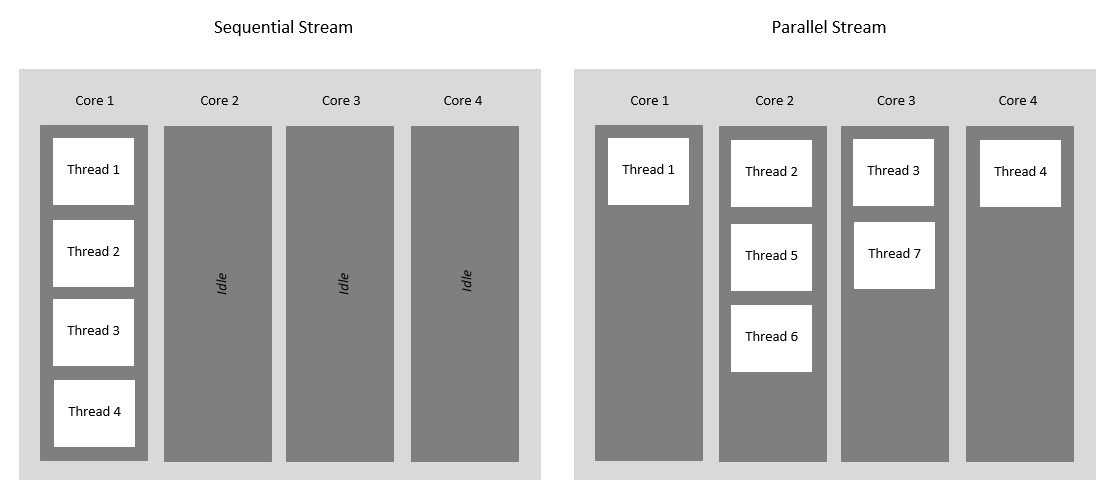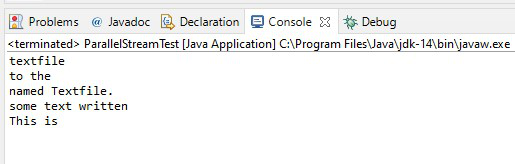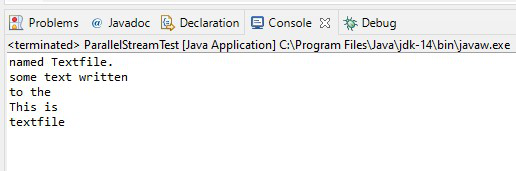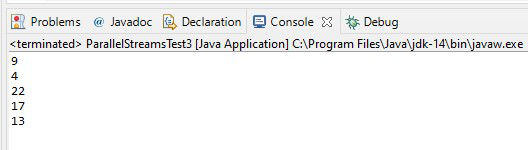GeeksforGeeks App
Open AppBrowser
Continue

# What is Java Parallel Streams?

Java Parallel Streams is a feature of Java 8 and higher, meant for utilizing multiple cores of the processor. Normally any java code has one stream of processing, where it is executed sequentially. Whereas by using parallel streams, we can divide the code into multiple streams that are executed in parallel on separate cores and the final result is the combination of the individual outcomes. The order of execution, however, is not under our control.

Therefore, it is advisable to use parallel streams in cases where no matter what is the order of execution, the result is unaffected and the state of one element does not affect the other as well as the source of the data also remains unaffected.### Why Parallel Streams?

Parallel Streams were introduced to increase the performance of a program, but opting for parallel streams isn’t always the best choice. There are certain instances in which we need the code to be executed in a certain order and in these cases, we better use sequential streams to perform our task at the cost of performance. The performance difference between the two kinds of streams is only of concern for large-scale programs or complex projects. For small-scale programs, it may not even be noticeable. Basically, you should consider using Parallel Streams when the sequential stream behaves poorly.

### Ways to Create Stream

There are two ways we can create which are listed below and described later as follows:

1. Using parallel() method on a stream
2. Using parallelStream() on a Collection

Method 1: Using parallel() method on a stream

The parallel() method of the BaseStream interface returns an equivalent parallel stream. Let us explain how it would work with the help of an example.

In the code given below, we create a file object which points to a pre-existent ‘txt’ file in the system. Then we create a Stream that reads from the text file one line at a time. Then we use the parallel() method to print the read file on the console. The order of execution is different for each run, you can observe this in the output. The two outputs given below have different orders of execution.

Example

## Java

 `// Java Program to Illustrate Parallel Streams``// Using parallel() method on a Stream`` ` `// Importing required classes``import` `java.io.File;``import` `java.io.IOException;``import` `java.nio.file.Files;``import` `java.util.stream.Stream;`` ` `// Main class``// ParallelStreamTest``public` `class` `GFG {`` ` `    ``// Main driver method``    ``public` `static` `void` `main(String[] args) ``throws` `IOException {`` ` `        ``// Creating a File object``        ``File fileName = ``new` `File(``"M:\\Documents\\Textfile.txt"``);`` ` `        ``// Create a Stream of string type``        ``// using the lines() method to``        ``// read one line at a time from the text file``        ``Stream text = Files.lines(fileName.toPath());`` ` `        ``// Creating parallel streams using parallel() method``        ``// later using forEach() to print on console``        ``text.parallel().forEach(System.out::println);`` ` `        ``// Closing the Stream``        ``// using close() method``        ``text.close();``    ``}``}`

Output:

1AOutput 1

1BOutput 2

Method 2: Using parallelStream() on a Collection

The parallelStream() method of the Collection interface returns a possible parallel stream with the collection as the source. Let us explain the working with the help of an example.

Implementation:

In the code given below, we are again using parallel streams but here we are using a List to read from the text file. Therefore, we need the parallelStream() method.

Example

## Java

 `// Java Program to Illustrate Parallel Streams``// using parallelStream() method on a Stream`` ` `// Importing required classes``import` `java.io.File;``import` `java.io.IOException;``import` `java.nio.file.Files;``import` `java.util.*;`` ` `// Main class``// ParallelStreamsTest``public` `class` `GFG {`` ` `    ``// Main driver method``    ``public` `static` `void` `main(String[] args)``        ``throws` `IOException``    ``{`` ` `        ``// Creating a File object``        ``File fileName``            ``= ``new` `File(``"M:\\Documents\\List_Textfile.txt"``);`` ` `        ``// Reading the lines of the text file by``        ``// create a List using readAllLines() method``        ``List text``            ``= Files.readAllLines(fileName.toPath());`` ` `        ``// Creating parallel streams by creating a List``        ``//  using readAllLines() method``        ``text.parallelStream().forEach(System.out::println);``    ``}``}`

Output:Output

My Personal Notes arrow_drop_up
Related Tutorials